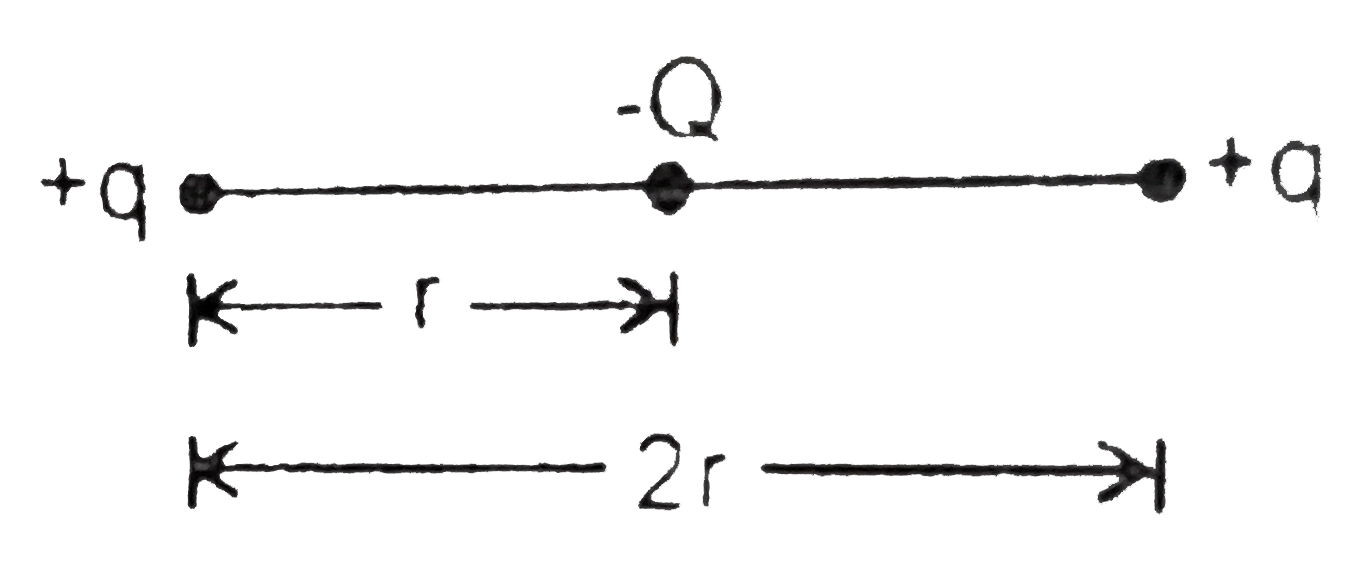# Figure shown an arrangement of three points charges. The total potential energy of this arrangement zero. The ratio qQ is :Khareedo DN Pro and dekho sari videos bina kisi ad ki rukaavat ke!

उत्तर

Step by step solution by experts to help you in doubt clearance & scoring excellent marks in exams.

Transcript

reference question is given figure shown in an arrangement of three point charge the total potential energy of the arrangement is zero the ratio of small cube By Capital Q ok so we know the potential energy is equal to K Q1 Q2 upon our ok so she potential of the system will be how much ke for this Kyon charge and this - future this one ke Kyon into minus 2 upon our + + q and this + q q and 2q upon to our distance upon this value for this q&q this will be a minus q q upon this distance
Aaoge so you also the system potential of the system is equal to how much take common here ke ok Cybertech common and this is equal to zero given ok so this will be how much few minor you upon our + this will be QQ upon 2 h + this will be how much minus q and this is small cube of 1 hour is equal to zero ok so from here this will be minus q q upon our + Q U upon to act plus minus 2 into 2 upon our is equal to zero ke will be if they will going right right hand side so this will be zero
ok so now take the common hear you EP 1 small ki open heart so this will be how much minus Q + this will be + you up on our and this will be - That is equal to zero ok so from this will be minus 2 minus plus you why to yah Kyon bhai hair 22 WhatsApp check our common Kyon so this will be too so is equal to zero ok Chopra maja -2 Q is equal to minus 1 upon 2 minus minus cancel ok so from here Q sorry we have to find the ratio of small Q upon capital q so you
upon this capital Q will be how much this is equal to 4.1 ratio will be how much 411 ok so sweet job Jano options are there so our correct of our correct answer will be for Y1 thank you

संबंधित वीडियो